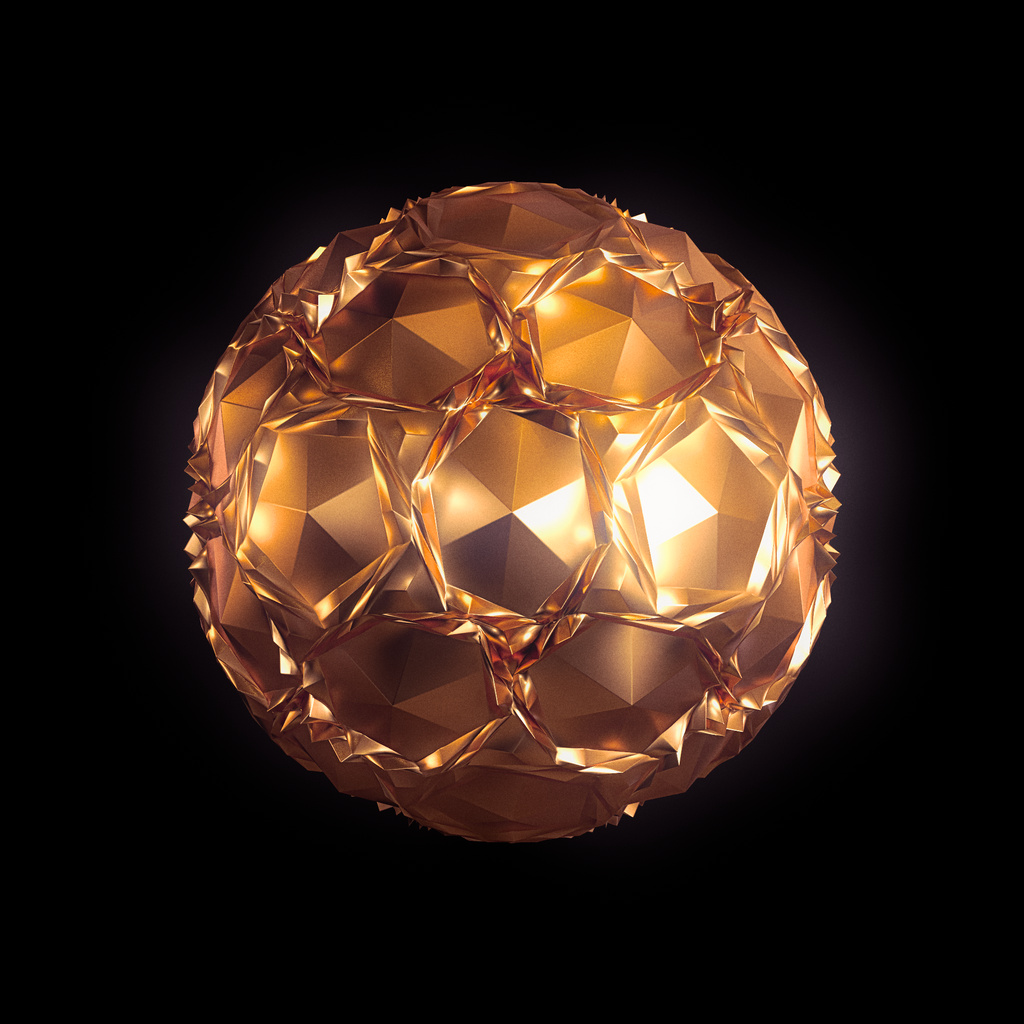# Conway-Hart Polyhedron Operations

This crate implements the Conway Polyhedron Operators and their extensions by George W. Hart and others.

The internal representation uses mesh buffers. These need furter preprocessing before they can be sent to a GPU but are almost fine to send to an offline renderer, as-is.

See the `playground` example for code on how to do either.

## Example

```use polyhedron_ops::Polyhedron;
use std::path::Path;

// Conway notation: gapcD
let polyhedron = Polyhedron::dodecahedron()
.chamfer(None, true)
.propellor(None, true)
.ambo(None, true)
.gyro(None, None, true)
.finalize();

// Export as ./polyhedron-gapcD.obj
polyhedron.write_to_obj(&Path::new("."), false);```

The above code starts from a dodecahedron and iteratively applies four operators.

The resulting shape is shown below.## Cargo Features

``````[dependencies]
polyhedron-ops = { version = "0.2.1", features = [ "bevy", "nsi", "obj" ] }
``````

## Modules

 prelude Re-exports commonly used types and traits.

## Structs

 Polyhedron

## Type Definitions

 Edge Edges EdgesSlice Face FaceSet Faces Float Normal Normals Point Points Vector VertexKey